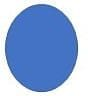Picture Vocabulary

# Geometric Shapes and Their DefinitionsGeometric shapes are the very basic part of primary classes in Mathematics. Kids learn different geometric shapes in their 1st, 2nd, and 3rd grades. This post will cover the names, images, and definitions of these geometric shapes in English.

PointA small dot-like shape to show the place of something is known as a point.

Segment

The part of a line b/w any two points on the line.

Ray

The half line is known as a ray.

Line

A line is a collection of continuous points in both directions.

Angle

An angle is formed when two rays share an endpoint.

Circle

The locus of equal distance point from the central point.

Oval

Any curve that looks like an egg.

Polygon

Any closed flat figure with three or more sides.

Acute angle

It is an angle whose measure is <90o.

Right Angle

An angle whose measure = 90o.

Obtuse angle

An angle whose measure is >90o.

Straight angle

It is an angle whose measure =180o.

Acute triangle

In this triangle, three interior angles are not greater than 90o in measure.

Scalene Triangle

A triangle with 3 sides that are not equal to each other.

Isosceles Triangle

A triangle has 2 compatible sides.

Right Triangle

A triangle with a 90o angle.

Equilateral Triangle

A triangle has 3 compatible sides & 3 compatible interior angles.

Perpendicular

2 co-planer lines that cross at right angles.

Parallel

Co-planer lines that do not cross each other at all.